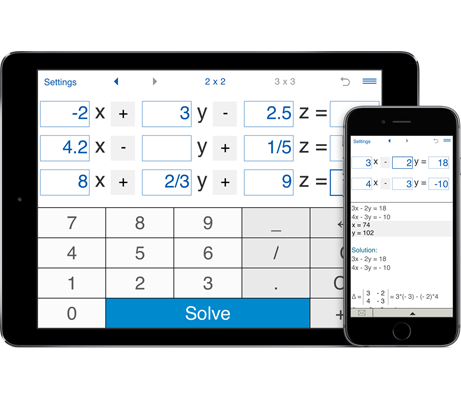# Solving systems of three linear equations in three variables

An easy to use calculator to solve systems of three linear equations in three variables 3x3.

## Solving systems of three linear equations in three variables

#### Systems of three linear equations in three variables 3x3

a11x1 + a12x2 + a13x3 = b1
a21x1 + a22x2 + a23x3 = b2
a31x1 + a32x2 + a33x3 = b3

where x1, x2, x3 are the unknowns, a11,..., a33 are the coefficients of the system, b1, b2, b3 are the constant terms

### 3x3 system of linear equations solver

System solver can be used for solving systems of three linear equations in three variables or checking the solutions of 3 x 3 systems of linear equations solved by hand.

To solve a system of three linear equations with three unknowns using the 3x3 system of equations solver, enter the coefficients of the three linear equations and click 'Solve'.

#### Solving a system of three linear equations in three variables using Cramer's rule

Example. Solving the system of three linear equations in three variables using Cramer's rule.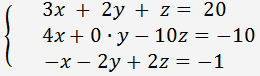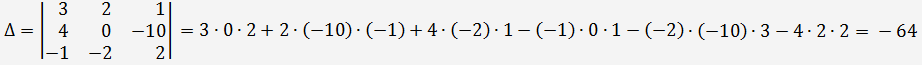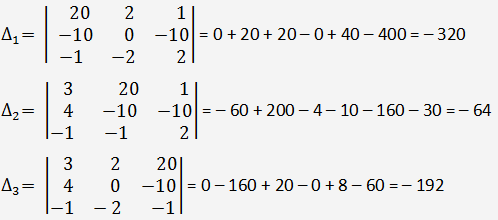By Cramer's rule: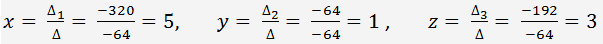#### Solving systems of three equations using Gaussian Elimination

Solving a system of linear equations using Gaussian Elimination

Example. Solving the system of three linear equations in three variables using Gaussian Elimination.Divide the first equation by 3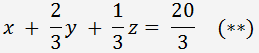Multiply (**) by 4 and add -1 times to the second equation, then multiply (**) by (-1) and add to the third equation. We get the following system: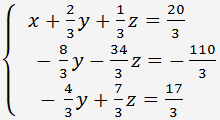Divide the second equation by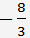and get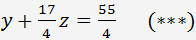Multiply (***) by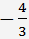and add -1 times to the third equation.

The system we get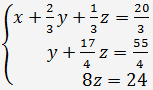From the third equation z=3. Substitute this to the second equation: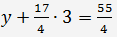=> y=1>

Substituting y and z to the first equation, we get x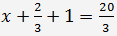=> x=5

x=5, y=1, z=3